# NCERT Solutions for Class 12 Chemistry Chapter 6 General Principles and processes of isolation of elements PDF - eSaral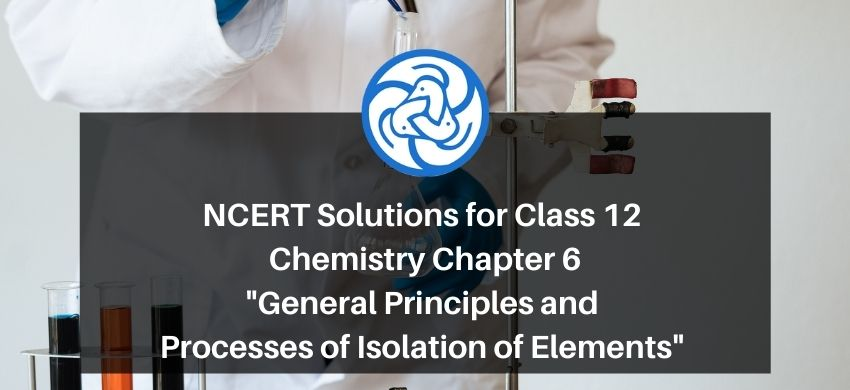# NCERT Solutions for Class 12 Chemistry Chapter 6 General Principles and processes of isolation of elements PDF

Hey, are you a class 12 student and looking for ways to download NCERT Solutions for Class 12 Chemistry Chapter 6 General Principles and processes of isolation of elements PDF? If yes. Then read this post till the end.

In this article, we have listed NCERT Solutions for Class 12 Chemistry Chapter 6 General Principles and processes of isolation of elements in PDF that are prepared by Kota’s top IITian Faculties by keeping Simplicity in mind.

If you want to learn and understand class 12 Chemistry Chapter 6 "General Principles and processes of isolation of elements" in an easy way then you can use these solutions PDF.

NCERT Solutions helps students to Practice important concepts of subjects easily. Class 12 Chemistry solutions provide detailed explanations of all the NCERT questions that students can use to clear their doubts instantly.

If you want to score high in your class 12 Chemistry Exam then it is very important for you to have a good knowledge of all the important topics, so to learn and practice those topics you can use eSaral NCERT Solutions.

In this article, we have listed NCERT Solutions for Class 12 Chemistry Chapter 6 General Principles and processes of isolation of elements PDF that you can download to start your preparations anytime.

So, without wasting more time Let’s start.

### Download NCERT Solutions for Class 12 Chemistry Chapter 6 General Principles and processes of isolation of elements PDF

Question 1. Which of the ores mentioned in the following table can be concentrated by magnetic separation method?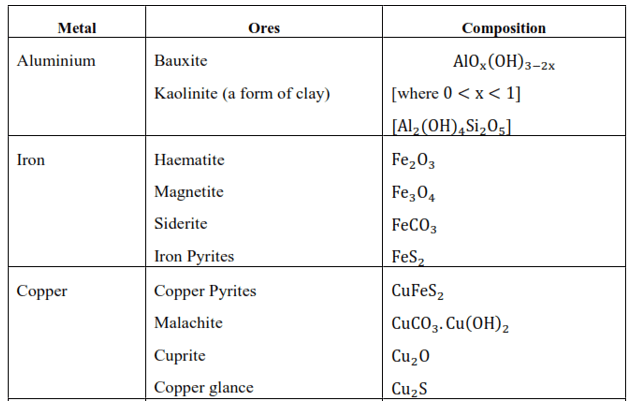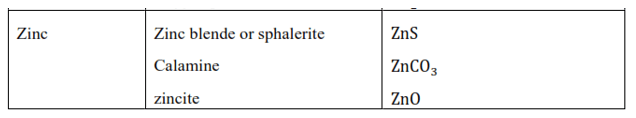Solution: The magnetic separation method is used when the ore or the gangue can be attracted by the magnetic field. Among the ores mentioned in the given table, the ores of iron such as haematite $\mathrm{Fe}_{2} \mathrm{O}_{3}$, magnetite $\mathrm{Fe}_{3} \mathrm{O}_{4}$, siderite $\mathrm{FeCO}_{3}$, and iron pyrites $\mathrm{FeS}_{2}$ can be separated by the process of magnetic separation.

Question 2. What is the significance of leaching in the extraction of aluminium?

Solution: In the extraction of aluminium, the significance of leaching is to concentrate pure alumina $\mathrm{Al}_{2} \mathrm{O}_{3}$ from bauxite ore.

Bauxite usually contains impurities such as silica, iron oxide, and titanium oxide. In the process of leaching, alumina is concentrated by digesting the powdered ore with a concentrated solution of $\mathrm{NaOH}$ at $473-523 \mathrm{~K}$ and $35-$ 36 bar. Under these conditions, alumina $\left(\mathrm{Al}_{2} \mathrm{O}_{3}\right)$ dissolves as sodium metaaluminate and silica $\mathrm{SiO}_{2}$ dissolves as sodium silicate leaving the impurities behind.

Question 3. The reaction,

$\mathrm{Cr}_{2} \mathrm{O}_{3}+2 \mathrm{Al} \rightarrow \mathrm{Al}_{2} \mathrm{O}_{3}+2 \mathrm{Cr}\left(\Delta \mathrm{G}^{\circ}=-421 \mathrm{~kJ} \mathrm{~mol}^{-1}\right)$

is thermodynamically feasible as is apparent from the Gibbs energy value. Why does it not take place at room temperature?

Solution. The change in Gibbs free energy is related to the equilibrium constant, $\mathrm{K}$ as

$\Delta \mathrm{G}=-\mathrm{RT} \ln \mathrm{K}$

At room temperature, all the reactants and the products at room temperature are solids. Equilibrium does not exist between the reactants and products. Hence the reaction does not take place at room temperature. A certain amount of energy (activation energy) is required for even the reactions which are thermodynamically stable.

Question 4. Is it true that under certain conditions, $\mathrm{Mg}$ can reduce $\mathrm{Al}_{2} \mathrm{O}_{3}$ and $\mathrm{Al}$ can reduce Mg0? What are those conditions?

Solution. Yes, it is true that magnesium (Mg) can reduce aluminium oxide $\left(\mathrm{Al}_{2} \mathrm{O}_{3}\right)$ and aluminium (Al) can also reduce magnesium oxide (MgO). According to the Ellingham diagram, we find that below $1350^{\circ} \mathrm{C}, \mathrm{Mg}$ can reduce aluminium oxide $\left(\mathrm{Al}_{2} \mathrm{O}_{3}\right)$ and when the temperature is above $1350^{\circ} \mathrm{C}, \mathrm{Al}$ can reduce magnesium oxide (Mg0).

Question 5. Explain why hydro-metallurgy can extract copper, but cannot extract zinc.

Solution. The reduction potential of copper is higher than that of zinc and iron. Thus, in hydro-metallurgy iron and zinc can be used to displace copper from its solution. $\mathrm{Fe}(\mathrm{s})+\mathrm{Cu}^{2+}(\mathrm{aq}) \rightarrow \mathrm{Fe}^{2+}(\mathrm{aq})+\mathrm{Cu}(\mathrm{s})$

But in order to displace a metal like zinc a metal more reactive than it is required e.g. $\mathrm{K}, \mathrm{Ca}, \mathrm{Mg}$ etc. The metals which are stronger reducing agent than zinc react with water to liberate hydrogen gas. Thus, hydro-metallurgy can extract copper but cannot extract zinc.

Question 6. What role does a depressant play in the process of froth floatation?

Solution. In the froth floatation process, the role of a depressant is to prevent certain types of substances from forming the froth with the air bubbles.

For example, $\mathrm{NaCN}$ is used as a depressant to separate two sulphide ores, PbS (Lead sulphide) and (Zinc sulphide). NaCN forms a complex, $\mathrm{Na}_{2}\left[\mathrm{Zn}(\mathrm{CN})_{4}\right]$ with zinc on the surface of zinc sulphide, thus preventing from forming the froth.

$4 \mathrm{NaCN}+\mathrm{Zns} \rightarrow \mathrm{Na}_{2}\left[\mathrm{Zn}(\mathrm{CN})_{4}\right]+\mathrm{Na}_{2} \mathrm{~S}$

Question 7. Why is it more difficult to extract copper from pyrites than it is to extract copper from its oxide ore?

Solution. $\Delta_{\mathrm{f}} \mathrm{G}$ (Gibbs free energy of formation) of copper (I) sulfite is less than that of hydrogen sulphite and carbon disulphite $\left(\mathrm{CS}_{2}\right)$. Therefore $\mathrm{C}$ and $\mathrm{H}_{2} \mathrm{~S}$ will not be able to reduce $\mathrm{Cu}_{2} \mathrm{~S}$ to $\mathrm{Cu}$.

Whereas $\Delta_{\mathrm{f}} \mathrm{G}$ of $\mathrm{Cu}_{2} \mathrm{O}$ is larger than carbon monoxide(CO). Therefore $\mathrm{C}$ can reduce copper (I) oxide to copper.

$\mathrm{C}(\mathrm{s})+\mathrm{Cu}_{2} \mathrm{O}(\mathrm{s}) \rightarrow 2 \mathrm{Cu}(\mathrm{s})+\mathrm{CO}(\mathrm{g})$

Therefore, it is more difficult to extract copper from copper pyrites than it is to extract from its oxide ore.

Question 8. Write notes on:

(a) Zone refining

(b) Column chromatography.

Solution. (a) Zone refining is based on the principle that impurities are more soluble in the molten state of metal than in solid metal. In zone refining, a circular mobile heater slowly moves over the impure metal rod. As the heater is removed along with the length of the rod, the pure metal crystallizes out of the melt and impurities pass into the adjacent molten zone. This process is repeated several times, causing impurities to get collected at one end of the rod. This impure end is then cut off and discarded.

Semiconductors and some metals of high purity like Boron, silicon, gallium etc. are purified using this method.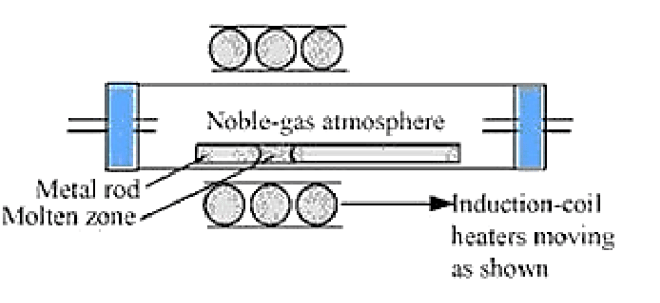(b) Column chromatography is a method used for purifying elements present in minute quantities. Column chromatography is based on the principle that various components of a mixture are adsorbed to different extents on an adsorbent. Chromatography includes two phases: Mobile phase and Stationary phase. A column of adsorbent e.g. aluminium oxide is used as the stationary phase. The mixture that is to be purified is dissolved in the mobile phase which can be either a liquid, gas or supercritical fluid. This is then poured onto the top of the column. The component which is more strongly adsorbed by the adsorbent takes more time to move through it than the component that is weakly adsorbed. This way the different components of the mixture are separated as they move through the stationary phase. There are several chromatographic techniques such as paper chromatography, column chromatography, gas chromatography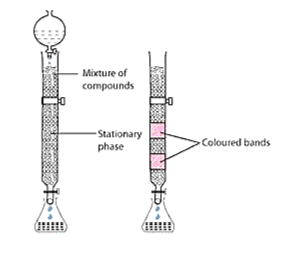Question 9. At $673 \mathrm{~K}$, which is a more effective reducing agent, $\mathrm{CO}$ or $\mathrm{C}$ ?

Solution. Carbon monoxide is a more effective reducing agent at $673 \mathrm{~K}$ because the value of $\Delta \mathrm{G}(\mathrm{C}, \mathrm{CO})$ is greater than the value of $\Delta \mathrm{G}\left(\mathrm{C}, \mathrm{CO}_{2}\right)$. This means that $\mathrm{CO}$ is more easily reduced to $\mathrm{CO}_{2}$ than $\mathrm{C}$ to $\mathrm{CO}$.

Question 10. In the process of electrolytic refining of copper what are the common elements in the anode mud? What is the reason for their presence?

Solution. During the electrolytic refining of copper - Silver, selenium, antimony, tellurium, platinum and gold are the common elements present in the anode mud.

As these metals are very less reactive, they are not affected during the purification method. Thus, they settle down under anode as anode mud.

Question 11. State the various reactions that take place inside the different zones of a blast furnace during iron extraction.

Solution. During the process of extraction of iron, iron oxide is reduced at different temperature ranges inside a blast furnace. The reactions taking place are:

At $500-800 \mathrm{~K}$ temperature zone:

$3 \mathrm{Fe}_{2} \mathrm{O}_{3}+\mathrm{CO} \rightarrow 2 \mathrm{Fe}_{3} \mathrm{O}_{4}+\mathrm{CO}_{2}$

$\mathrm{Fe}_{2} \mathrm{O}_{3}+\mathrm{CO} \rightarrow 2 \mathrm{FeO}+\mathrm{CO}_{2}$

At $850 \mathrm{~K}$ temperature zone:

$\mathrm{Fe}_{3} \mathrm{O}_{4}+\mathrm{CO} \rightarrow 3 \mathrm{FeO}+\mathrm{CO}_{2}$

At $900-1500 \mathrm{~K}$ temperature zone:

$\mathrm{FeO}+\mathrm{CO} \rightarrow \mathrm{Fe}+\mathrm{CO}_{2}$

$\mathrm{CO}_{2}+\mathrm{C} \rightarrow 2 \mathrm{CO}$

Above $1570 \mathrm{~K}$ temperature zone:

$\mathrm{C}+\mathrm{O}_{2} \rightarrow \mathrm{CO}_{2}$

$\mathrm{FeO}+\mathrm{C} \rightarrow \mathrm{Fe}+\mathrm{CO}$

$\mathrm{CaCO}_{3}(\mathrm{~s}) \rightarrow \mathrm{CaO}(\mathrm{s})+\mathrm{CO}_{2}(\mathrm{~g})$

$\mathrm{SiO}_{2}+\mathrm{CaO} \rightarrow \mathrm{CaSiO}_{3}($ slag $)$

Question 12. Write the chemical reactions involved in zinc extraction from zinc blende.

Solution. The steps involved in the extraction of zinc from zinc blende are:

(1) Concentration of ore

Firstly, zinc blende is crushed and the concentration of ore is done by froth floatation method.

(2) Roasting(Conversion to oxide)

In this step, $\mathrm{ZnS}$ is roasted in the presence of an excess of air at about $1200 \mathrm{~K}$ in a furnace. This results in the formation of zinc oxide.

$2 \mathrm{ZnS}+3 \mathrm{O}_{2} \rightarrow 2 \mathrm{Zn} 0+2 \mathrm{SO}_{2}$ (3) Reduction(Extraction of Zn from $\mathrm{ZnO}$ ):

Zinc oxide obtained after roasting is mixed with powdered coke and then heated to get zinc.

$\mathrm{Zn} \mathrm{O}+\mathrm{C} \rightarrow \mathrm{Zn}+\mathrm{CO}$

(4) Electrolytic Refining:

In electrolytic refining, impure zinc is taken as the anode while the cathode is a pure copper strip. The electrolyte is an acidified solution of zinc sulphate $\left(\mathrm{ZnSO}_{4}\right)$ with dilute sulfuric acid. On passing current, electrolysis takes place in which pure zinc is deposited on the copper strip.

Question 13. What role does silica play in copper metallurgy?

Solution. Copper pyrites are converted to a mixture of $\mathrm{Cu}_{2} \mathrm{O}$ and $\mathrm{FeO}$ during roasting.

$2 \mathrm{CuFeS}_{2}+\mathrm{O}_{2} \rightarrow \mathrm{Cu}_{2} \mathrm{~S}+2 \mathrm{FeS}+\mathrm{SO}_{2}$

$2 \mathrm{Cu}_{2} \mathrm{~S}+3 \mathrm{O}_{2} \rightarrow 2 \mathrm{Cu}_{2} \mathrm{O}+2 \mathrm{SO}_{2}$

$2 \mathrm{FeS}+3 \mathrm{O}_{2} \rightarrow 2 \mathrm{Fe} 0+2 \mathrm{SO}_{2}$

Silica is used in the metallurgy of copper to remove the iron oxide obtained during the process of roasting as slag. $\mathrm{SiO}_{2}$ and $\mathrm{FeO}$ form $\mathrm{FeSiO}_{3}$ (slag) which floats on the molten matter.

$\mathrm{Fe} \mathrm{O}+\mathrm{SiO}_{2} \rightarrow \mathrm{FeSiO}_{3}($ slag $)$

Question 14. "Chromatography", What do you understand by this term?

Solution. Chromatography is a term used for a range of laboratory techniques for the separation and purification of mixtures. The word has been derived from Greek words; 'chroma' meaning 'colour' and 'graphein' meaning 'to write'. There are various chromatographic techniques like column chromatography, paper chromatography, gas chromatography, etc.

Question 15. What criterion is followed for the selection of the stationary phase in chromatography?

Solution. The stationary phase during chromatography is selected in such a way that the components of the sample have different solubilities in the phase. Different components have different rates of movement through the stationary phase and hence can be separated from each other. The stationary phase used is immobile and immiscible.

Question 16. Write about a technique used to refine nickel?

Solution. Mond's process is the technique used to refine nickel. In this process, heat is supplied to nickel in the presence of carbon monoxide to produce nickel tetracarbonyl(volatile complex).

$\mathrm{Ni}+4 \mathrm{CO} \stackrel{330-350 \mathrm{~K}}{\longrightarrow} \mathrm{Ni}(\mathrm{CO})_{4}$

Nickel tetracarbonyl

The nickel tetracarbonyl obtained is then decomposed by heating it at a higher temperature $(450-470 \mathrm{~K})$ to form pure nickel.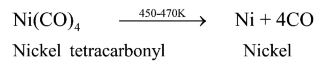Question 17. How is alumina separated from silica in a bauxite ore associated with silica? Provide equations, if present.

Solution. Alumina separated from silica in a bauxite ore associated with silica is as follows:

Firstly, a concentrated $\mathrm{NaOH}$ solution is used to digest the powdered ore at a temperature of $473-523 \mathrm{~K}$ and at a pressure of $35-36$ bar. This causes leaching of alumina $\left(\mathrm{Al}_{2} \mathrm{O}_{3}\right)$ and silica $\left(\mathrm{SiO}_{2}\right)$ as sodium aluminate and sodium silicate respectively leaving the impurities behind.

$\mathrm{Al}_{2} \mathrm{O}_{3}(\mathrm{~s})+2 \mathrm{NaOH}(\mathrm{aq})+3 \mathrm{H}_{2} \mathrm{O}(\mathrm{l}) \rightarrow 2 \mathrm{Na}\left[\mathrm{Al}(\mathrm{OH})_{4}\right](\mathrm{aq})$

and

$\mathrm{SiO}_{2}+2 \mathrm{NaOH}(\mathrm{aq}) \rightarrow \mathrm{Na}_{2} \mathrm{SiO}(\mathrm{aq})+\mathrm{H}_{2} \mathrm{O}(\mathrm{l})$

To neutralize the aluminate present in the solution, carbon dioxide gas is passed through the solution we obtained. This causes the sodium meta-aluminate to precipitate as hydrated alumina.

$2 \mathrm{Na}\left[\mathrm{Al}(\mathrm{OH})_{4}\right](\mathrm{aq})+\mathrm{CO}_{2}$

$\rightarrow \mathrm{Al}_{2} \mathrm{O}_{3} \cdot \mathrm{xH}_{2} \mathrm{O}(\mathrm{s})+2 \mathrm{NaHCO}_{3}(\mathrm{aq})$

Sodium silicate present cannot be precipitated so it is filtered. The hydrated alumina is dried, heated and filtered to obtain the pure alumina.

$\mathrm{Al}_{2} \mathrm{O}_{3} \cdot \mathrm{xH}_{2} \mathrm{O}(\mathrm{s}) \rightarrow \mathrm{Al}_{2} \mathrm{O}_{3}(\mathrm{~s})$

$+\mathrm{xH}_{2} \mathrm{O}(\mathrm{g})[1470 \mathrm{~K}]$

Question 18. Differentiate between 'calcination' and 'roasting' with the help of examples.

Solution.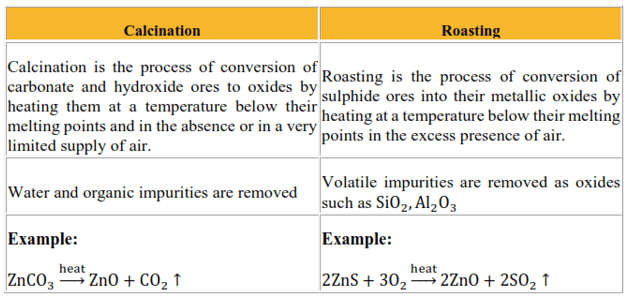Question 19. What is the difference between 'cast iron' and 'pig iron'?

Solution. Cast iron is made by melting pig iron and coke using a hot air blast. It has low levels of carbon in it $(3 \%)$. Cast iron is brittle and extremely hard unlike pig iron obtained from blast furnace.

Pig iron is the iron obtained from blast furnaces. It is around $4 \%$ carbon and contains many impurities like Si, P, S, Mn in smaller amounts.

Question 20. What is the difference between "ores" and "minerals"?

Solution. The naturally occurring substances in the form of which metals occur in the earth's crust along with impurities are called minerals.

Ores are rocks and minerals from which metals can be conveniently obtained and is economically viable.

For example, aluminium occurs in the earth's crust both in the form of bauxite and clay. Therefore both bauxite and clay are minerals that contain aluminium. But out of these two minerals, aluminium can be only conveniently and economically extracted from the bauxite. Therefore bauxite is the ore.

Question 21. Why is copper matte put in converters lined with silica?

Solution: Copper matte consists of $\mathrm{Cu}_{2} \mathrm{~S}$ and some $\mathrm{FeS}$. When a hot blast of air is blown through a molten matte placed in a silica lined converter, FeS of the matte oxidizes to Fe0. This FeO combines with $\mathrm{SiO}_{2}$ (silica) to produce $\mathrm{FeSiO}_{3}$ (slag).

$2 \mathrm{FeS}+3 \mathrm{O}_{2} \rightarrow 2 \mathrm{FeO}+2 \mathrm{SO}_{2}$

$\mathrm{FeO}+\mathrm{SiO}_{2} \rightarrow \mathrm{FeSiO}_{3}$

When all of the iron has been removed as slag $\mathrm{Cu}_{2} \mathrm{~S}$ is oxidized to $\mathrm{Cu}_{2} \mathrm{O}$ which then undergoes a reaction with $\mathrm{Cu}_{2} \mathrm{~S}$ to give copper metal.

$2 \mathrm{Cu}_{2} \mathrm{O}+\mathrm{Cu}_{2} \mathrm{~S} \rightarrow 6 \mathrm{Cu}+\mathrm{SO}_{2}$

Question 22. In the metallurgy of aluminium, what role does Cryolite play?

Solution. Cryolite $\left(\mathrm{Na}_{3} \mathrm{AlF}_{6}\right)$ has two basic roles in the metallurgy of aluminium

1. The melting point of the solution is decreased from $2323 \mathrm{~K}$ to $1140 \mathrm{~K}$.

2. It increases the electrical conductivity of the mixture.

3. How is leaching done in when dealing with low grade copper ores?

Solution: For low-grade copper ores, bacteria or acids are used in the presence of air to leach the copper. In this method, copper goes into the solution as $\mathrm{Cu}^{2+}$ ions.

$\mathrm{Cu}(\mathrm{s})+2 \mathrm{H}^{+}(\mathrm{aq})+\frac{1}{2} \mathrm{O}_{2}(\mathrm{~g})$

$\rightarrow \mathrm{Cu}^{2+}(\mathrm{aq})+2 \mathrm{H}_{2} \mathrm{O}(\mathrm{l})$

This solution so obtained is then treated with $\mathrm{H}_{2}$ or scrap iron to obtain copper metal.

$\mathrm{Cu}^{2+}(\mathrm{aq})+\mathrm{H}_{2}(\mathrm{~g}) \rightarrow \mathrm{Cu}(\mathrm{s})+2 \mathrm{H}^{+}$

Question 23: The choice of a reducing agent in a particular case depends on

thermodynamic factor. How far do you agree with this statement? Support

Solution.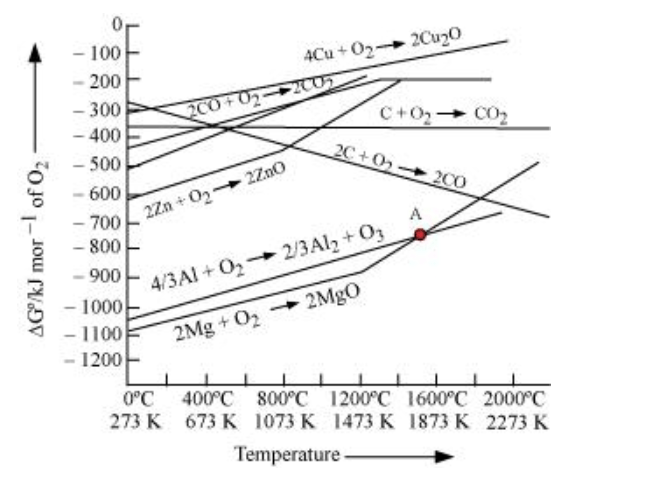The above figure is a plot of Gibbs energy $\left(\Delta \mathrm{G}^{\Theta}\right) \mathrm{vs}$. $\mathrm{T}$ for formation of some oxides. It can be observed from the above graph that a metal can reduce the oxide of other metals, if the standard free energy of formation $\left(\Delta_{\mathrm{f}} \mathrm{G}^{\circ}\right)$ of the oxide of the former is more negative than the latter. For example, since ${ \left.\Delta_{\mathrm{f}} \mathrm{G}_{\left(\mathrm{Al}, \mathrm{A} l_{2}\right.}^{\mathrm{O}}, \mathrm{O}_{3}\right)}$ is more negative than ${ }^{\triangle \mathrm{f} \mathrm{G}}^{\mathrm{O}}$

cannot reduce $\mathrm{Al}_{2} \mathrm{O}_{3}$. Similarly, $\mathrm{Mg}$ can reduce $\mathrm{ZnO}$ to $\mathrm{Zn}$, but $\mathrm{Zn}$ cannot reduce $\mathrm{MgO}$ because

$\Delta_{\mathrm{f}} \mathrm{G}_{ }^{\mathrm{O}}(\mathrm{Mg}, \mathrm{Mg} \mathrm{O})$ is more negative than $\left.\Delta \mathrm{f} \mathrm{G}_{(\mathrm{Zn},}^{\Theta} \mathrm{ZnO}\right)$.

Question 24. Why isn't zinc oxide reduction (using carbon monoxide) used to extract zinc?

Solution. The standard Gibbs free energy of formation of $\mathrm{Zn} \mathrm{O}$ from $\mathrm{Zn}$ is lower than that of $\mathrm{CO}_{2}$ from CO. So CO cannot reduce $\mathrm{Zn} \mathrm{O}$ to $\mathrm{Zn}$. Therefore zinc oxide reduction using carbon monoxide is not used to extract zinc.

Question 25. $\quad \Delta_{\mathrm{f}} G^{\theta}$ has a value of $-540 \mathrm{~kJ} \mathrm{~mol}^{-1}$ for $\mathrm{Cr}_{2} \mathrm{O}_{3}$ formation and $-827 \mathrm{~kJ} \mathrm{~mol}^{-1}$ for $\mathrm{Al}_{2} \mathrm{O}_{3}$ formation. Can $\mathrm{Cr}_{2} \mathrm{O}_{3}$ be reduced by Al?

Solution. $\mathrm{Cr}_{2} \mathrm{O}_{3}$ has a higher $\Delta_{\mathrm{f}} \mathrm{G}^{0}\left(-540 \mathrm{~kJ} \mathrm{~mol}^{-1}\right)$ than $\mathrm{Al}_{2} \mathrm{O}_{3}\left(-827 \mathrm{~kJ} \mathrm{~mol}^{-1}\right)$. Thus, Al can reduce $\mathrm{Cr}_{2} \mathrm{O}_{3}$ to $\mathrm{Cr}$. Alternatively,

$2 \mathrm{Al}+\left(\frac{3}{2}\right) \mathrm{O}_{2} \rightarrow \mathrm{Al}_{2} \mathrm{O}_{3}$...(i)

$2 \mathrm{Cr}+\left(\frac{3}{2}\right) \mathrm{O}_{2} \rightarrow \mathrm{Cr}_{2} \mathrm{O}_{3}$...(ii)

Subtracting equation (ii) from (i), we get

$2 \mathrm{Al}+\mathrm{Cr}_{2} \mathrm{O}_{3} \rightarrow \mathrm{Al}_{2} \mathrm{O}_{3}+2 \mathrm{Cr} ; \Delta_{\mathrm{f}} \mathrm{G}^{0}$

$=-827-(-540)=-287 \mathrm{~kJ} \mathrm{~mol}^{-1}$ As $\Delta_{\mathrm{f}} G^{0}$ is negative for the reduction reaction of $\mathrm{Cr}_{2} \mathrm{O}_{3}$ by Al, the reaction can take place.

Question 26. Which one is better at reducing $\mathrm{ZnO}, \mathrm{C}$ or $\mathrm{CO}_{2} ?$

Solution.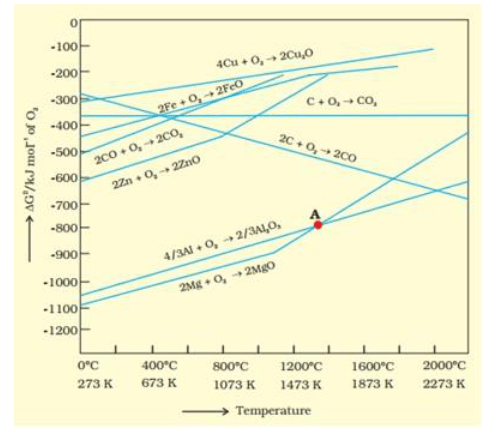ZnO is reduced to $\mathrm{Zn}$ at around $1673 \mathrm{~K}$. From the figure above, we observe that beyond $1073 \mathrm{~K}$ the Gibbs free energy of formation $\left(\Delta_{\mathrm{f}} G^{0}\right)$ of $\mathrm{CO}$ from $\mathrm{C}$ and beyond $1273 \mathrm{~K}$, the Gibbs free energy of formation of $\mathrm{CO}_{2}$ from $\mathrm{C}$ is less than the Gibbs free energy of formation of $\mathrm{ZnO}$. Thus, $\mathrm{C}$ can reduce $\mathrm{Zn} \mathrm{O}$ to $\mathrm{Zn}$. However, the Gibbs free energy of formation of $\mathrm{CO}_{2}$ from $\mathrm{CO}$ is greater than the Gibbs free energy of formation of $\mathrm{ZnO}$. Thus, $\mathrm{CO}$ is not able to reduce $\mathrm{ZnO}$. Therefore, out of $\mathrm{C}$ and $\mathrm{CO}, \mathrm{C}$ is a better reducing agent than $\mathrm{CO}$ for $\mathrm{ZnO}$.

Question 27. In some particular case, the thermodynamic factor dictates the choice of the reducing agent. To what extent do you believe this statement to be true? Provide two examples to back your opinion.

Solution.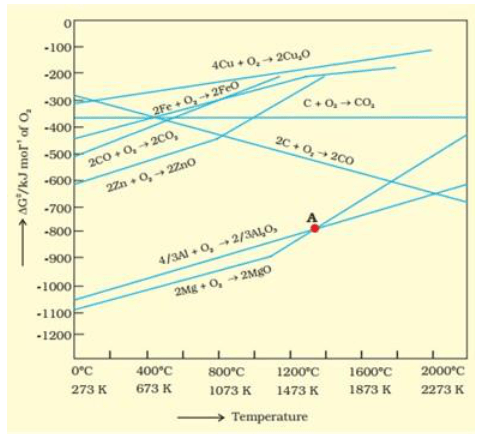From the above Ellingham diagram, we observe that metals for which the standard free energy of formation of their oxides is more negative can reduce the metal oxides for which the standard free energy of formation of their respective oxides is less negative.

Example 1: $\Delta_{\mathrm{f}} \mathrm{G}^{\theta}\left(\mathrm{Al}, \mathrm{Al}_{2} \mathrm{O}_{3}\right)$ is more negative than $\Delta_{\mathrm{f}} \mathrm{G}^{\theta}\left(\mathrm{Cu}, \mathrm{Cu}_{2} \mathrm{O}\right)$, thus $\mathrm{Al}$ can readily reduce $\mathrm{Cu}_{2} \mathrm{O}$ to $\mathrm{Cu}$ but it is not possible for $\mathrm{Cu}$ to reduce $\mathrm{Al}_{2} \mathrm{O}_{3}$.

Example 2: $\Delta_{\mathrm{f}} G^{\theta}(\mathrm{Mg}, \mathrm{MgO})$ is more negative than $\Delta_{\mathrm{f}} \mathrm{G}^{\theta}(\mathrm{Zn}, \mathrm{ZnO})$, thus it is not possible for $\mathrm{Zn}$ to reduce $\mathrm{Mg} 0$ but $\mathrm{Mg}$ can reduce $\mathrm{Zn} \mathrm{O}$ to $\mathrm{Zn}$.

Question 28. What are the processes that give chlorine as a by-product? What happens when an aqueous of $\mathrm{NaCl}$ is electolyzed?

Solution. Chlorine is obtained as a byproduct during the electrolysis of $\mathrm{NaCl}$ in Down's process. In this process a fused mixture of $\mathrm{CaCl}_{2}$ and $\mathrm{NaCl}$ is electrolyzed at a temperature of $873 \mathrm{~K}$. Sodium is liberated at the cathode and $\mathrm{Cl}_{2}$ is liberated at the anode.

$\mathrm{NaCl}(\mathrm{l}) \stackrel{\text { electrolysis }}{\longrightarrow} \mathrm{Na}^{+}+\mathrm{Cl}^{-}$

Cathode: $\mathrm{Na}^{+}(\mathrm{melt})+\mathrm{e}^{-} \rightarrow \mathrm{Na}($ solid $)$

Anode: $\mathrm{Cl}^{-}($melt $) \rightarrow \mathrm{Cl}(\mathrm{g})+\mathrm{e}^{-}$

$2 \mathrm{Cl}(\mathrm{g}) \rightarrow \mathrm{Cl}_{2}(\mathrm{~g})$

When an aqueous solution of $\mathrm{NaCl}$ is subjected to electrolysis, $\mathrm{Cl}_{2}$ is discharged at the anode and at the cathode, $\mathrm{H}_{2}$ is obtained (in place of $\mathrm{Na}$ ). This is due to the fact that the standard reduction potential of $\mathrm{H}_{2} \mathrm{O}\left(\mathrm{E}^{0}=-0.83 \mathrm{~V}\right)$ is less negative than that of $\mathrm{Na}\left(\mathrm{E}^{\circ}=-2.71 \mathrm{~V}\right) .$ Thus, $\mathrm{H}_{2} \mathrm{O}$ will get reduced at the cathode thereby discharging $\mathrm{H}_{2}$.

$\mathrm{NaCl}(\mathrm{aq}) \stackrel{\text { electrolysis }}{\longrightarrow} \mathrm{Na}^{+}(\mathrm{aq})+\mathrm{Cl}^{-}(\mathrm{aq})$

Cathode: $2 \mathrm{H}_{2} \mathrm{O}(\mathrm{l})+2 \mathrm{e}^{-}$

$\rightarrow \mathrm{H}_{2}(\mathrm{~g})+2 \mathrm{OH}^{-1}(\mathrm{aq})$

Anode: $2 \mathrm{Cl}(\mathrm{g}) \rightarrow \mathrm{Cl}_{2}(\mathrm{~g})$

Question 29. What role does a graphite rod play in the electrometallurgy of aluminium?

Solution. In the electrometallurgy of aluminium, Graphite rod acts as anode and graphite lined iron acts as the cathode. The $\mathrm{O}_{2}$ liberated due to electrolysis reacts with the graphite at the anode to liberate $\mathrm{CO}_{2}$ and $\mathrm{CO}$. The oxygen liberated would have reacted with the aluminium during the electrolysis to form $\mathrm{Al}_{2} \mathrm{O}_{3}$ if any other metal besides graphite was used. This would have resulted in the wastage of aluminium and as graphite is cheaper than aluminium it is more expendable.

Question 30. What are the principles of refining a metal by the following processes:

(a) Zone refining

(b) Electrolytic refining

(c) Vapour phase refining

Solution. (a) Zone refining:

This method is based on the principle that the impurities are more soluble in the melt than in the solid state of the metal.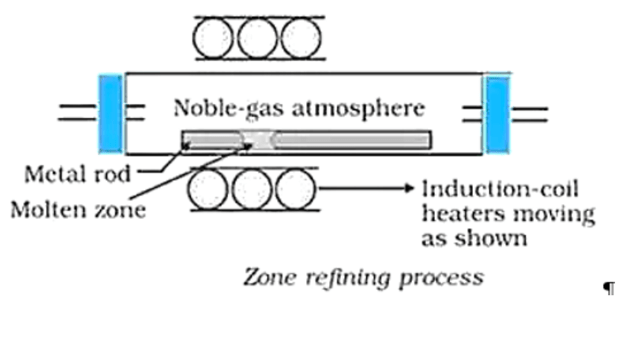(b) Electrolytic refining:

Electrolytic refining works on the principle of refining impure metals by the use of electricity. In this process, the impure metal is placed as the anode and a strip of pure metal is made as the cathode. Soluble salt of the same metal in a solution is taken as the electrolyte. When an electric current is passed, metal ions from the electrolyte are deposited at the cathode as pure metal and the impure metal from the anode dissolves into the electrolyte in the form of ions. The impurities present in the impure metal gets collected below the anode as the anode mud.

(c) Vapour phase refining:

In this method, the metal is converted into its volatile compound which is collected and decomposed to give pure metal. Thus, two requirements of this process are:

(i) the metal should form a volatile compound with an available reagent, and

(ii) the volatile compound should be easily decomposable so that the recovery of the metal is easy.

Question 31. Under what conditions could Al reduce MgO?

Solution. Above $1350^{\circ} \mathrm{C}, \mathrm{Al}$ can reduce MgO. This is because, at temperatures above $1350^{\circ} \mathrm{C}$, the standard Gibbs free energy formation of $\mathrm{Al}_{2} \mathrm{O}_{3}$ from $\mathrm{Al}$ is less than that of $\mathrm{Mg} \mathrm{O}$ from $\mathrm{Mg}$.